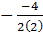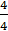### Sample Problem

Find the vertex of the following quadratic function

y = 2x2 – 4x + 5

Vertex: (, )

#### Solution

In order to find the vertex, we first find the axis of symmetry

x === 1

Now, we know that the x coordinate of the vertex is 1, so we can plug this in and solve for the y coordinate

y = 2(1)2 – 4(1) + 5 = 2 – 4 + 5 = 3

So the vertex is at (1, 3)